Deep Learning

# BERT Transformers – How Do They Work?

March 29, 2021### BERT Transformers Are Revolutionary But How Do They Work?

BERT, introduced by Google in 2018, was one of the most influential papers for NLP. But it is still hard to understand. BERT stands for Bidirectional Encoder Representations from Transformers.

In this article, we will go a step further and try to explain BERT Transformers. BERT is one of the most popular NLP models that utilizes a Transformer at its core and which achieved State of the Art performance on many NLP tasks including Classification, Question Answering, and NER Tagging when it was first introduced.

Specifically, we will try to go through the highly influential BERT paperPre-training of Deep Bidirectional Transformers for Language Understanding while keeping the jargon to a minimum.

### What is BERT?

In simple words, BERT is an architecture that can be used for a lot of downstream tasks such as question answering, Classification, NER etc. One can assume a pre-trained BERT as a black box that provides us with H = 768 shaped vectors for each input token(word) in a sequence. Here, the sequence can be a single sentence or a pair of sentences separated by the separator [SEP] and starting with a token [CLS]. We will get into explaining these tokens in more detail in later stages.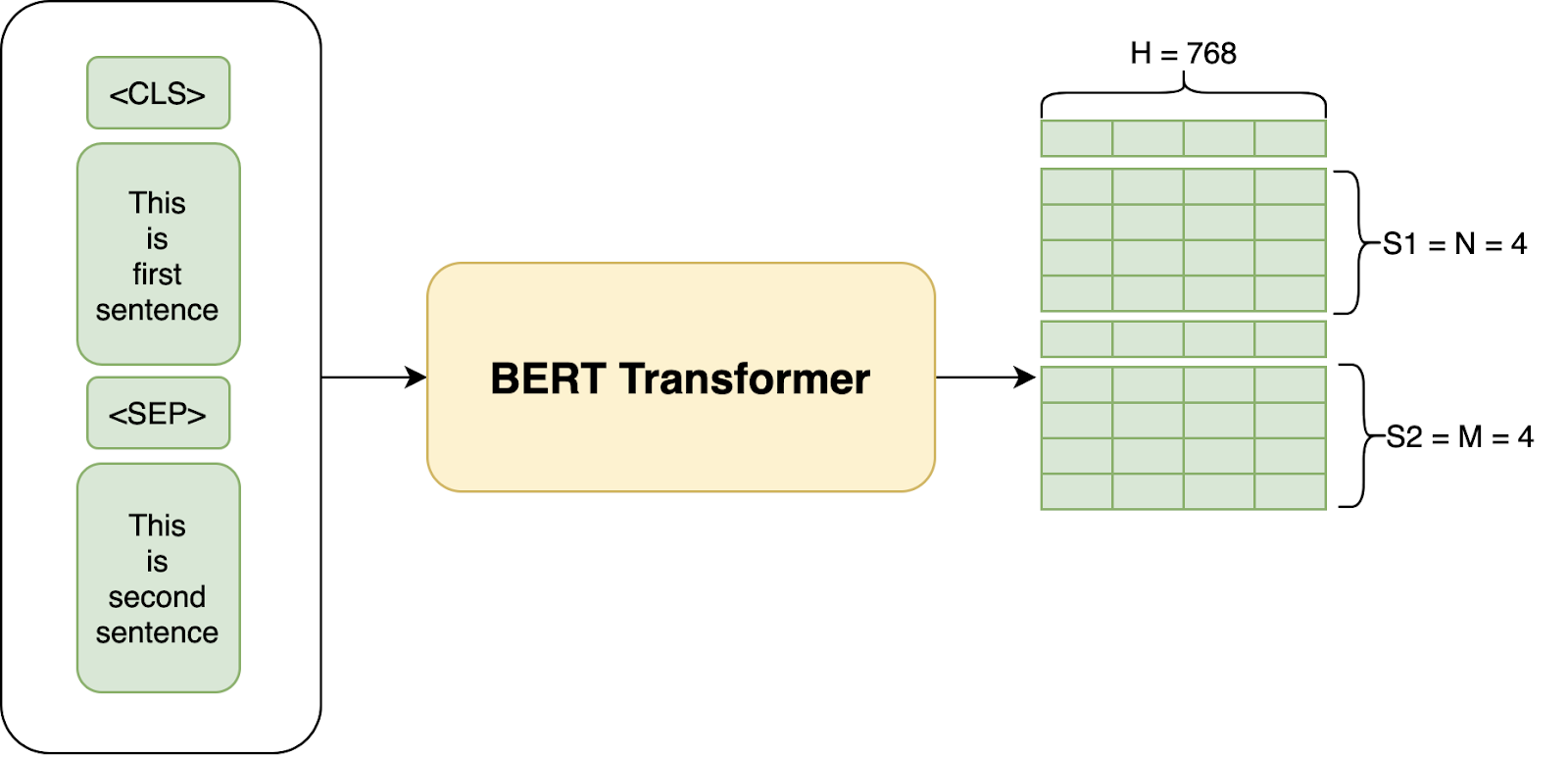A very High view of BERT — We get a 768 sized vector for all words in our input sentence.

### What is the Use of a BERT Blackbox?

A BERT model works like how most Deep Learning models for ImageNet work

First, we train the BERT model on a large corpus (Masked LM Task), and then we finetune the model for our own task which could be classification, Question Answering or NER, etc. by adding a few extra layers at the end.

For example, we would train BERT first on a corpus like Wikipedia (Masked LM Task) and then Fine Tune the model on our own data to do a classification task like classifying reviews as negative or positive or neutral by adding a few extra layers. In practice, we just use the output from the [CLS] token for the classification task. So, the whole architecture for fine-tuning looks like this: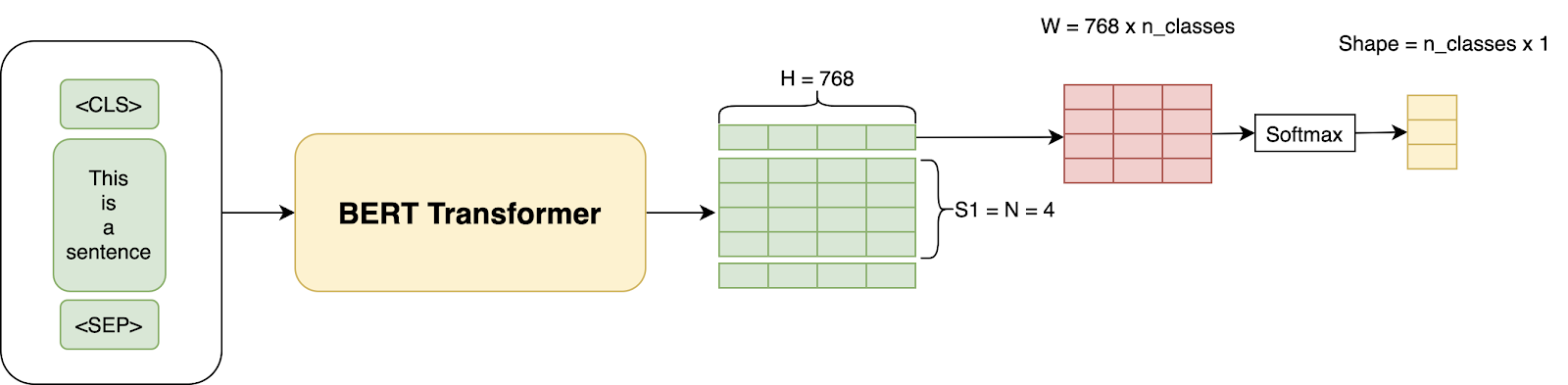We just use the [CLS] Token output for classification along with some added Linear and Softmax Layers.

In other similarly published articles on transformers, all Deep Learning is just Matrix Multiplication, where we just introduce a new W layer having a shape of (H x num_classes = 768 x 3) and train the whole architecture using our training data and Cross-Entropy loss on the classification.

We could have also acquired the sentence features through the last layer and then just run a Logistic regression classifier on top or take an average of all the outputs and then run a logistic regression on top. There are many possibilities, and what works best will depend on the data for the task.

In the above example, we explained how you could do Classification using BERT. In pretty much similar ways, one can also use BERT for Question Answering and NER based Tasks. We will get to the architectures used for various tasks in a few sections below.

Interested in an AI workstation that can handle natural language processing?
View deep learning workstations starting at \$3,700

### How is this Different Than Embedding?

This is a great question. Essentially, BERT just provides us with contextual-bidirectional embeddings.

Let’s unpack what that means:

• Contextual: The embeddings of a word are not static. That is, they depend on the context of words around it. So in a sentence like "one bird was flying below another bird", the two embeddings of the word "bird" will be different.
• Bi-Directional: While directional models in the past like LSTM’s read the text input sequentially (left-to-right or right-to-left), the Transformer actually reads the entire sequence of words at once and thus is considered bidirectional.

So, for a sentence like "BERT model is awesome." the embeddings for the word "model" will have context from all the words "BERT", "Awesome", and "is".

### How Does It Work?

Now that we understand the basics, we will divide this section into three major parts: Architecture, Inputs, and Training.

#### 1. Architecture

This is the most simple part to understand if you’re familiar with this post on Transformers. BERT is essentially just made up of stacked up encoder layers.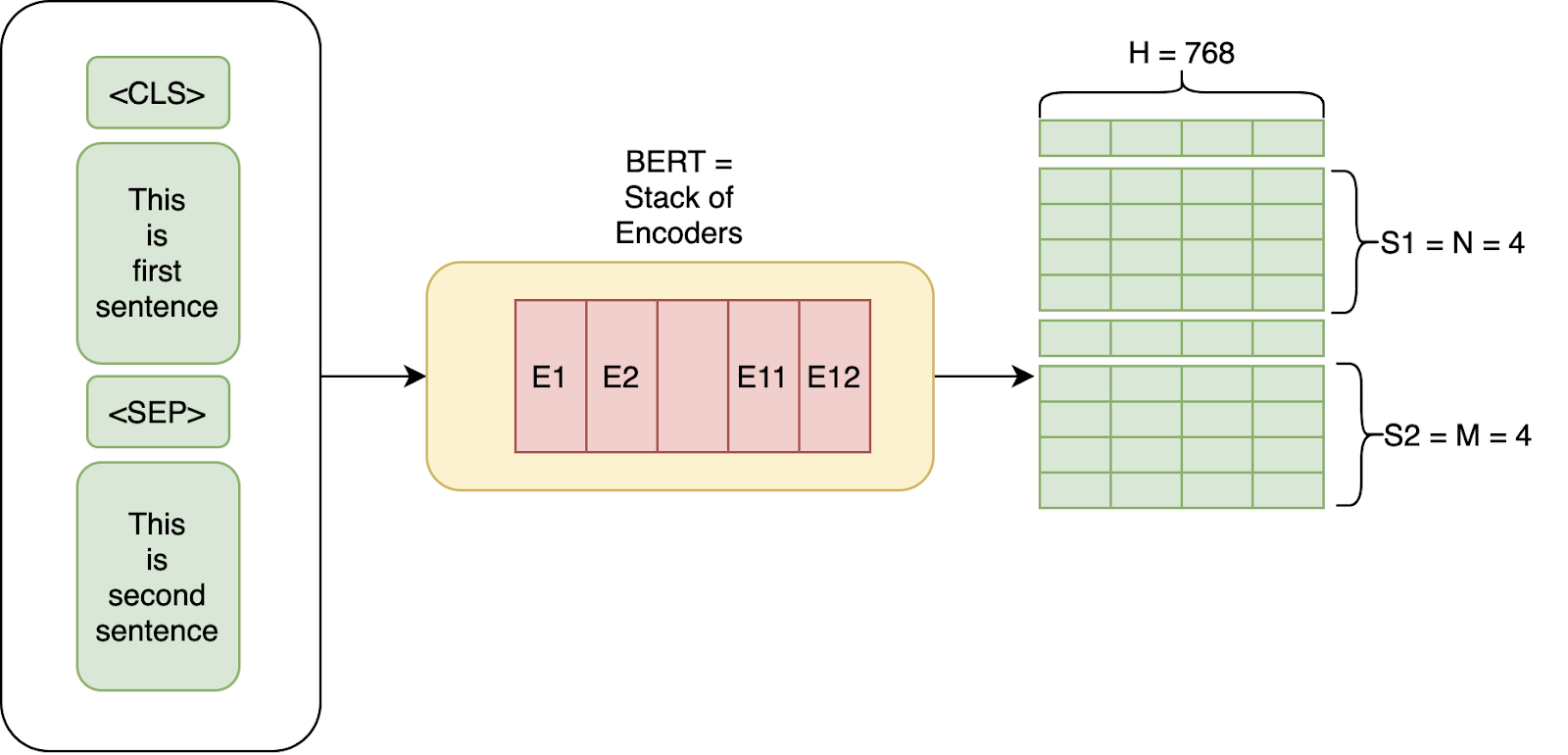In the paper, the authors have experimented with two models:

• BERT Base: Number of Layers L=12, Size of the hidden layer, H=768, and Self-attention heads, A=12 with Total Parameters=110M
• BERT Large: Number of Layers L=24, Size of the hidden layer, H=1024, and Self-attention heads, A=16 with Total Parameters=340M

#### 2. Training Inputs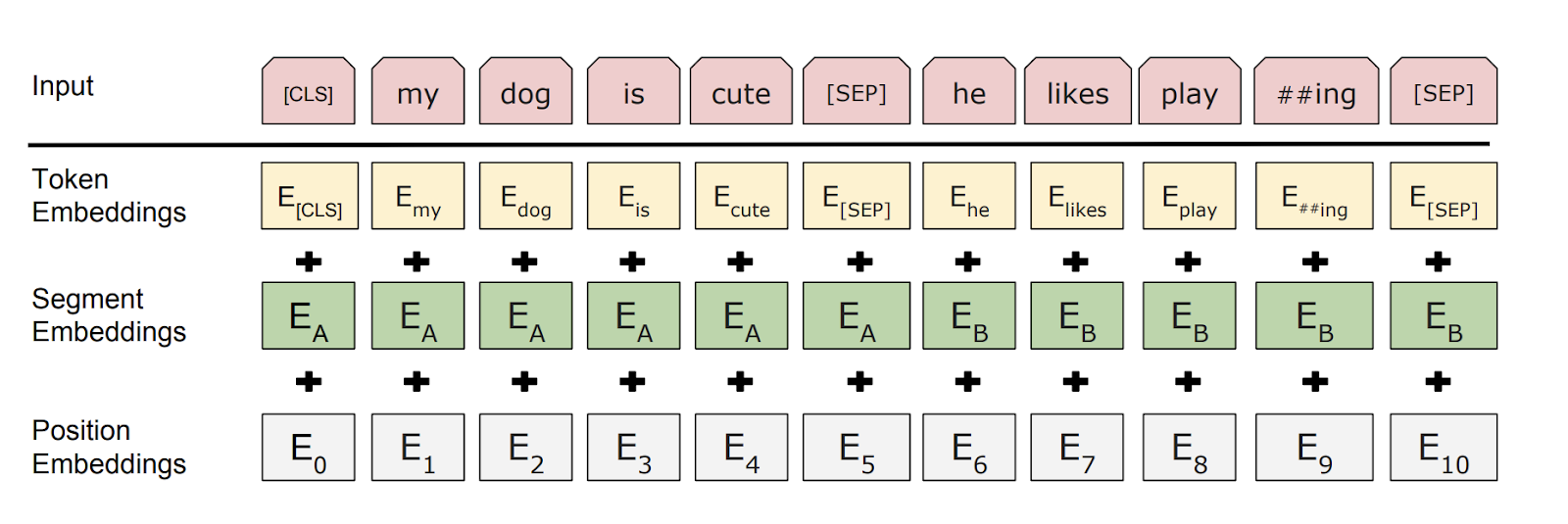Inputs to BERT. Source

We give inputs to BERT using the above structure. The input consists of a pair of sentences, called sequences, and two special tokens: [CLS] and [SEP].

So, in this example, for two sentences “my dog is cute” and “he likes playing”, BERT first uses wordpiece tokenization to convert the sequence into tokens and adds the [CLS] token in the start and the [SEP] token in the beginning and end of the second sentence, so the input is: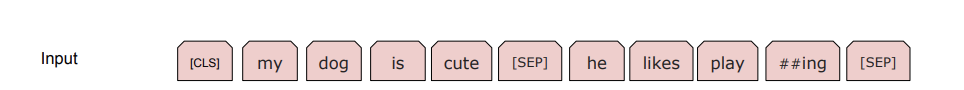The wordpiece tokenization used in BERT necessarily breaks words like playing into “play” and “##ing”. This helps in two ways:

• It helps limit the size of Vocabulary as we don’t have to keep the various forms of words like playing, plays, player etc. in our vocabulary.
• It helps us with out-of-vocab words. For example, if plays don’t occur in the vocabulary, we might still have embeddings for play and ##s

Token Embeddings: We then get the Token embeddings by indexing a Matrix of size 30000x768(H). Here, 30000 is the Vocab length after wordpiece tokenization. The weights of this matrix would be learned while training.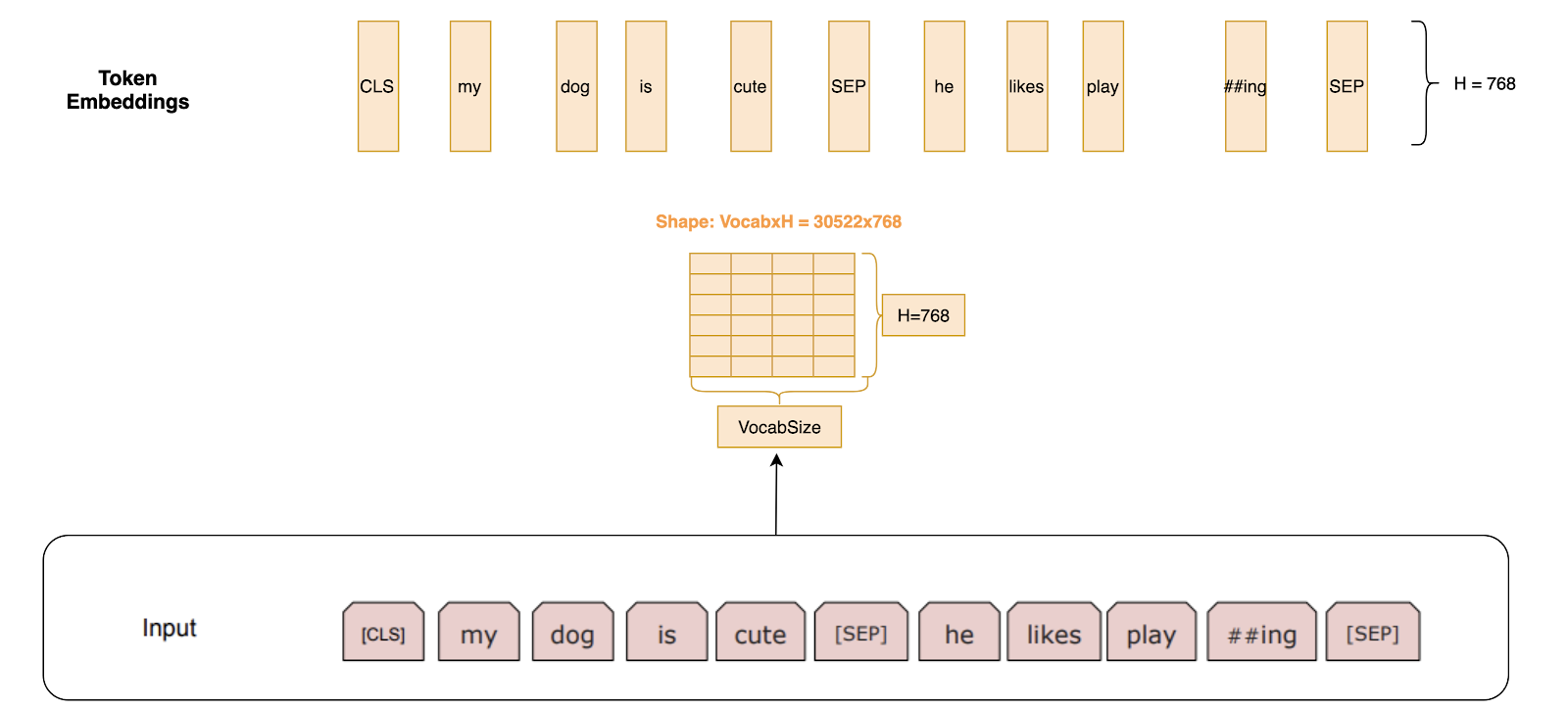Token Embeddings come by indexing a matrix of size VocabxH.

Segment Embeddings: For tasks such as question answering, we should specify which segment this sentence is from. These are either all 0 vectors of H length if the embedding is from sentence 1, or a vector of 1’s if the embedding is from sentence 2.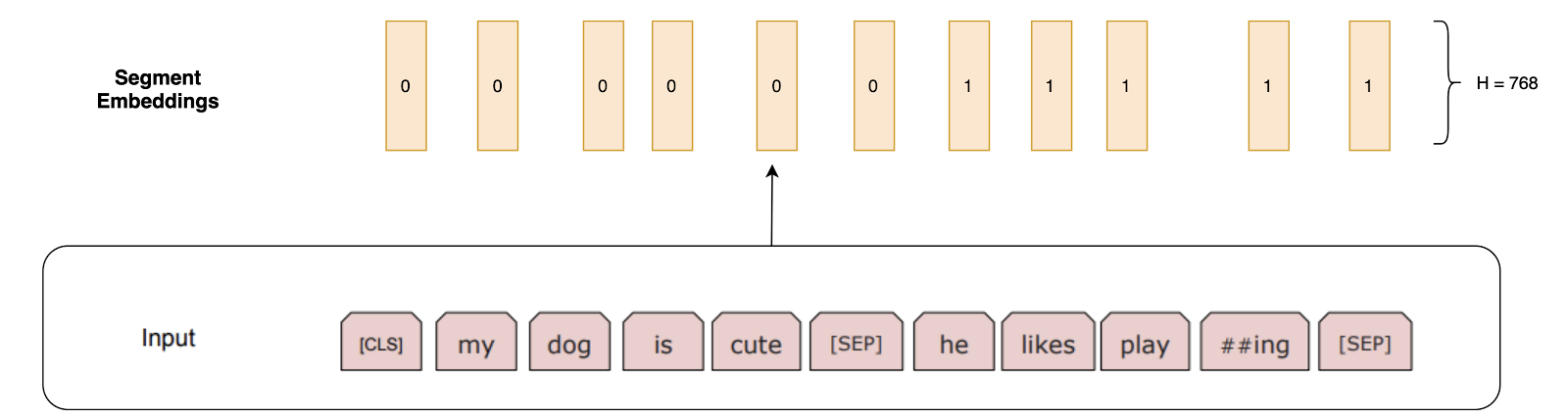Segment embedding are all 0's or all 1’s vector specifying 1st Sentence or 2nd Sentence.

Position Embeddings: These are the embeddings used to specify the position of words in the sequence, the same as we did in the transformer architecture. So we essentially have a constant matrix with some preset pattern. This matrix has the number of columns as 768. The first row of this matrix is the embedding for token [CLS], the second row is embedding for the word “my”, the third row is embedding for the word “dog” and so on.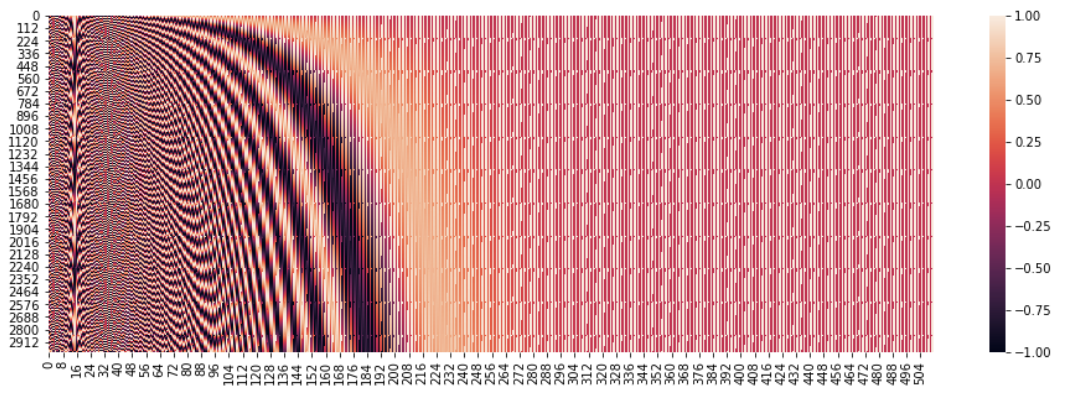Patterns are used to specify word position.

So the Final Input given to BERT is Token Embeddings + Segment Embeddings + Position Embeddings.

We finally reach the most interesting part of BERT here, as this is where most of the novel concepts are introduced. We will try to explain these concepts by going through various architecture attempts and finding faults with each of them to arrive at the final BERT architecture.

Attempt 1: So, for example, if we set up BERT training as below: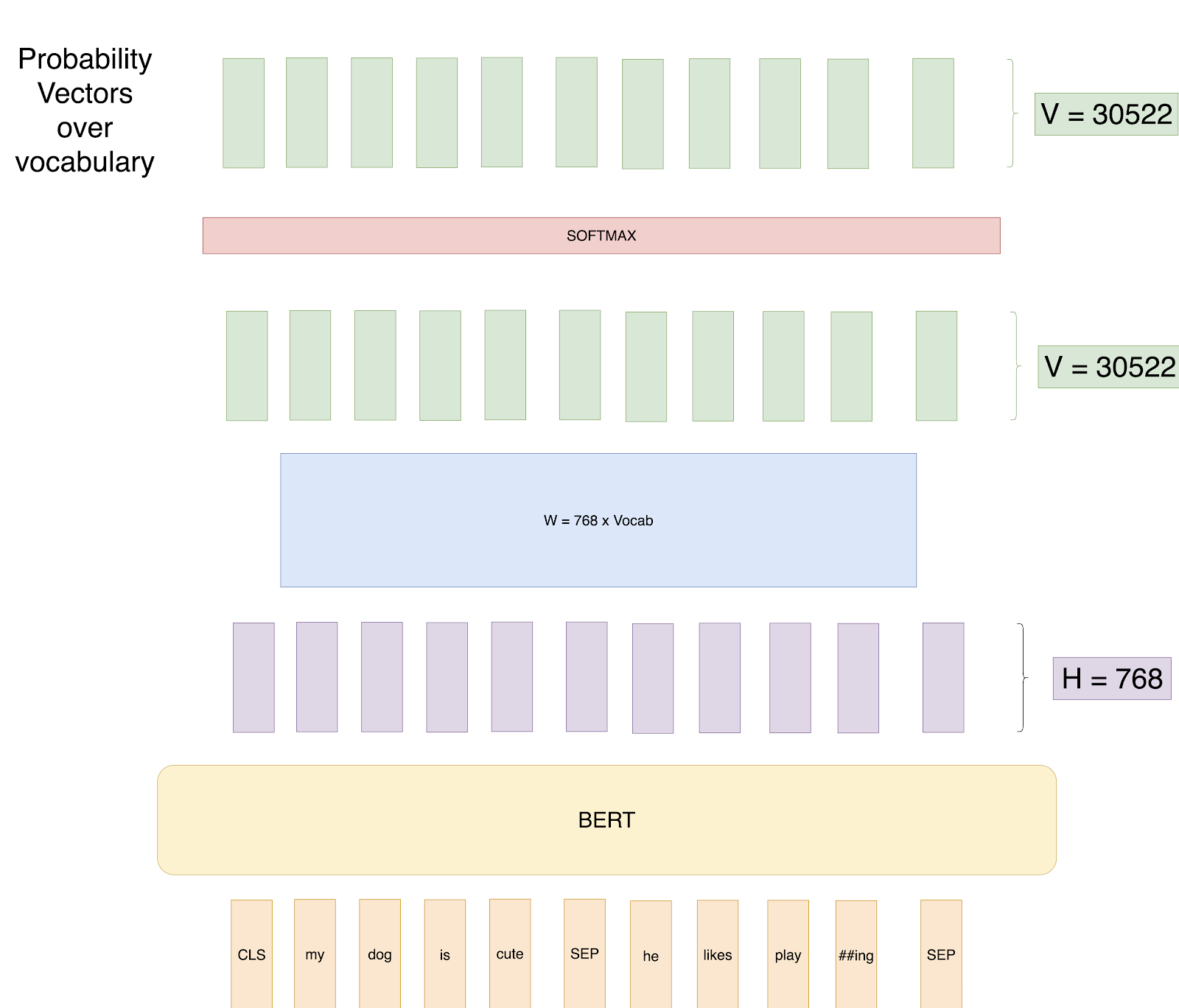Attempt 1: Predict all words.

We try to predict each word of the input sequence using our training data with Cross-Entropy loss.

Can you guess a potential problem with this approach?

The problem is that the learning task is trivial.

The network knows beforehand what it has to predict, and it can thus easily learn weights to reach a 100% classification accuracy.

Attempt 2: Masked LM: This starts the beginning of the paper's approach to overcoming the previous approach's problem. We mask 15% random words in each training input sequence and just predict output for those words. In Pictorial terms: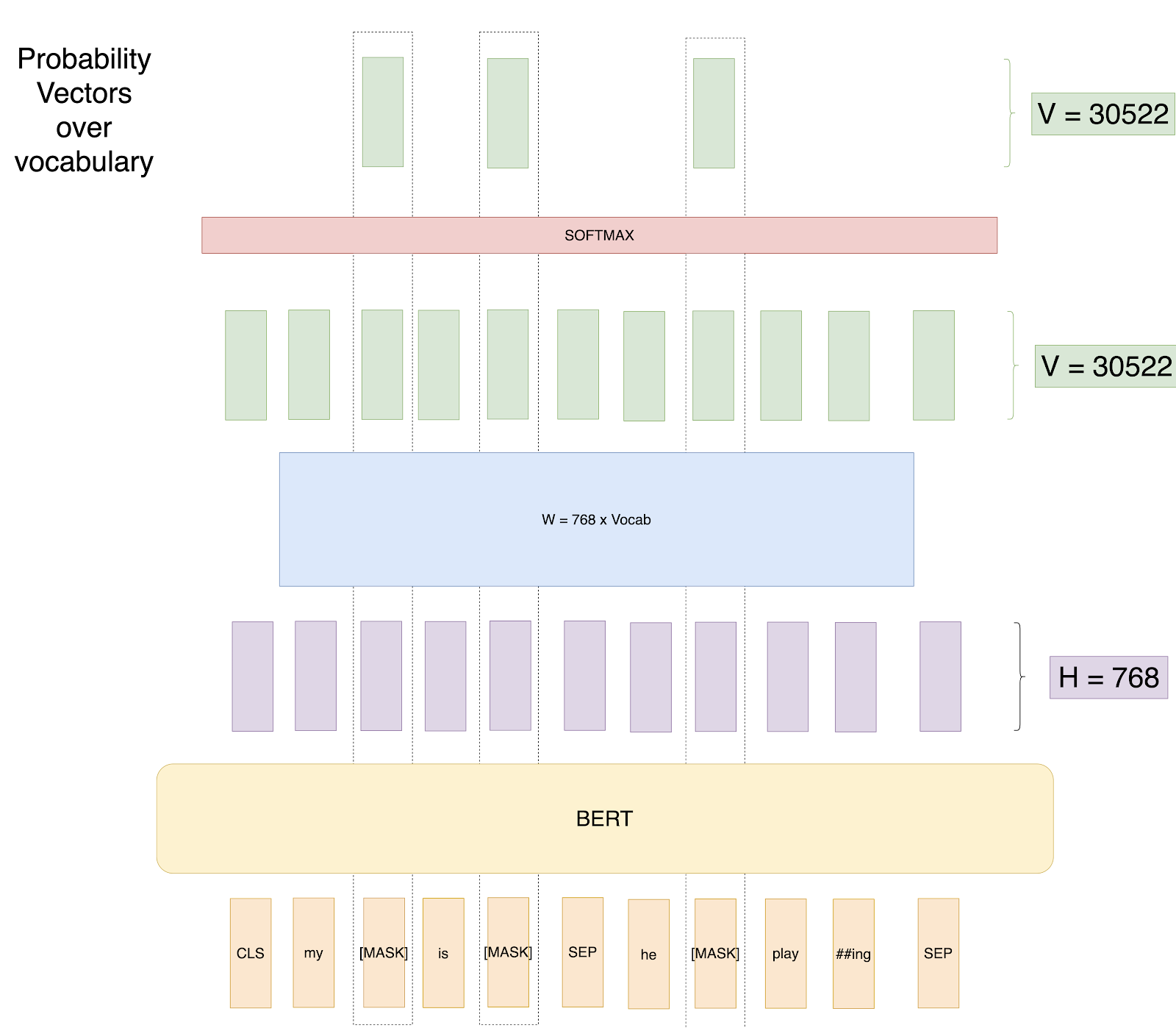Attempt 2: Predict only masked words.

Thus the loss gets calculated only over masked words. So now the model learns to predict words it hasn’t seen while seeing all the context around those words.

Please note, we have masked 3 words here even when we should mask just 1 word as 15% of 8 is 1 to explain in this toy example.

Can you find the problem with this approach?

This model has essentially learned that it should predict good probabilities for only the [MASK] token. That is at the prediction time or at the fine-tuning time when this model will not get [MASK] as input; the model won’t predict good contextual embeddings.

Attempt 3: Masked LM with random Words:

In this attempt, we will still mask 15% of the positions. But we will replace any word in 20% of those masked tokens by some random word. We do this because we want to let the model know that we still want some output when the word is not a [MASK] token. So if we have a sequence of length 500, we will mask 75 tokens(15% of 500), and in those 75 tokens, 15 tokens(20 % of 75) would be replaced by random words. Pictorially, here we replace some of the masks by random words.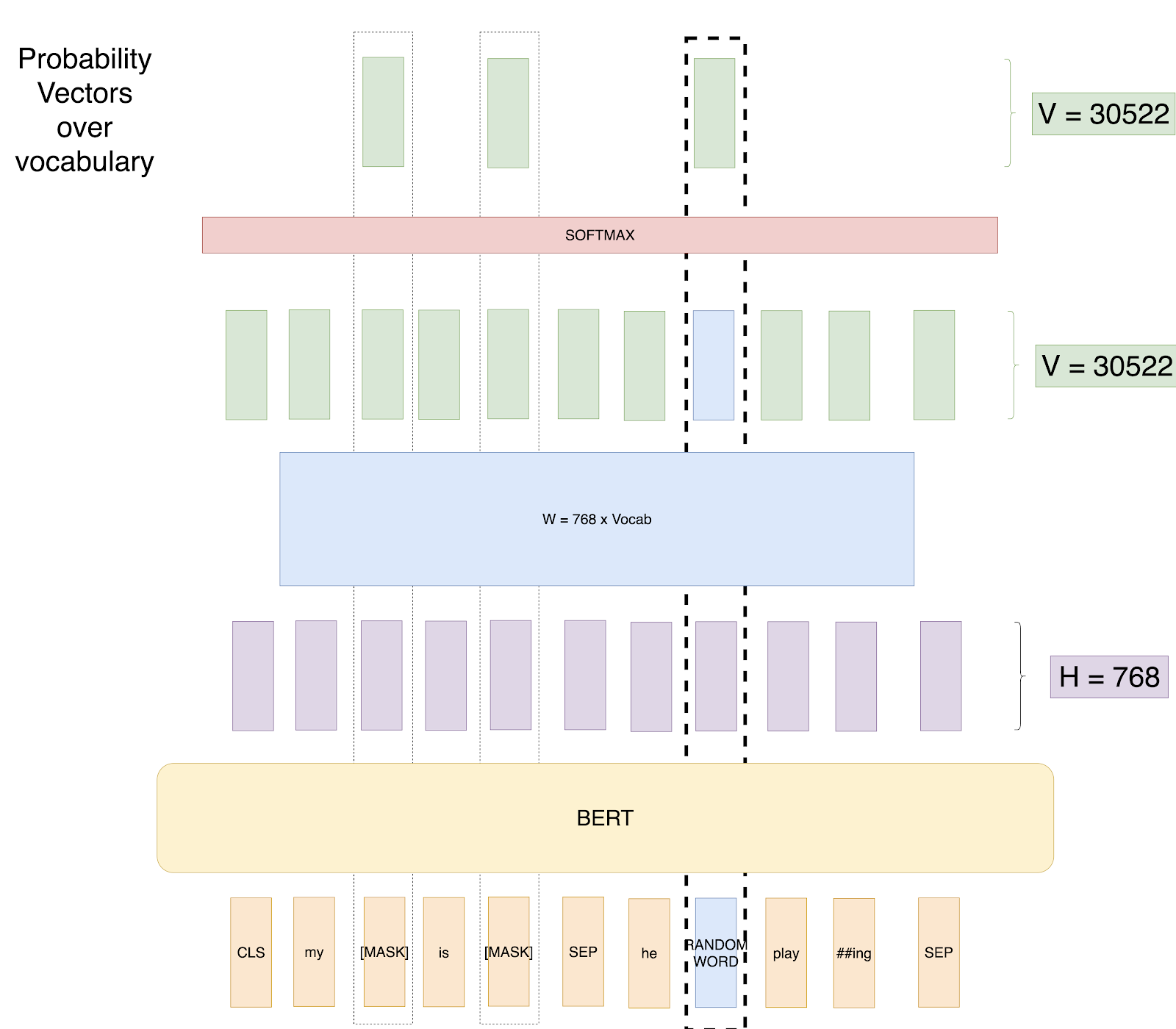Attempt 3: Predict masked words and Random Words.

Advantage: The network will still work with any word now.

Problem: The network has learned that Input Word is never equal to the Output word. That is the output vector at the position of “random word” would never be “random word.”

To solve this problem, the authors suggest the below training setup.

The training data generator chooses 15% of the token positions at random for prediction. If the i-th token is chosen, we replace the i-th token with (1) the [MASK] token 80% of the time (2) a random token 10% of the time (3) the unchanged i-th token 10% of the time

So if we have a sequence of length 500, we will mask 75 tokens(15% of 500), and in those 75 tokens, 7 tokens(10 % of 75) would be replaced by random words, and 7 tokens (10% of 75) will be used as it is. Pictorially, we replace some of the masks with random words and replace some of the masks by actual words.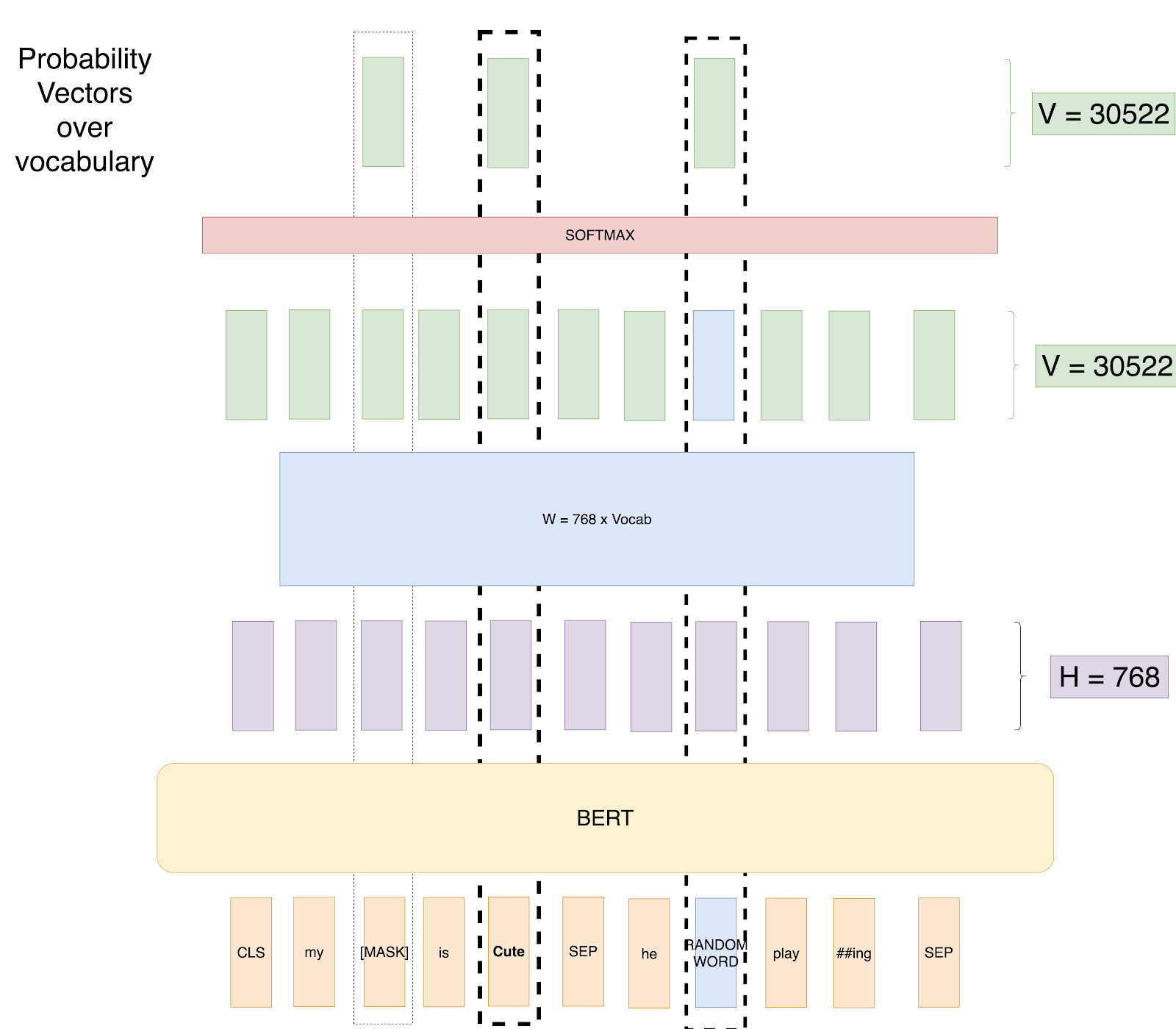So, now we have the best setup where our model doesn’t learn any unsavory patterns.

But what if we keep only Mask + Unmask Setup? The model will learn that whenever the word is present, just predict that word.

From the BERT paper:

Many important downstream tasks such as Question Answering (QA) and Natural Language Inference (NLI) are based on understanding the relationship between two sentences, which is not directly captured by language modeling. In order to train a model that understands sentence relationships, we pre-train for a binarized next sentence prediction task that can be trivially generated from any monolingual corpus.

So, now we understand the Masked LM task, BERT Model also has one more training task which goes in parallel while Training Masked LM task. This task is called Next Sentence Prediction (NSP). So while creating the training data, we choose the sentences A and B for each training example such that 50% of the time B is the actual next sentence that follows A (labelled as IsNext), and 50% of the time it is a random sentence from the corpus (labelled as NotNext). We then use the CLS token output to get the binary loss which is also back propagated through the network to learn the weights.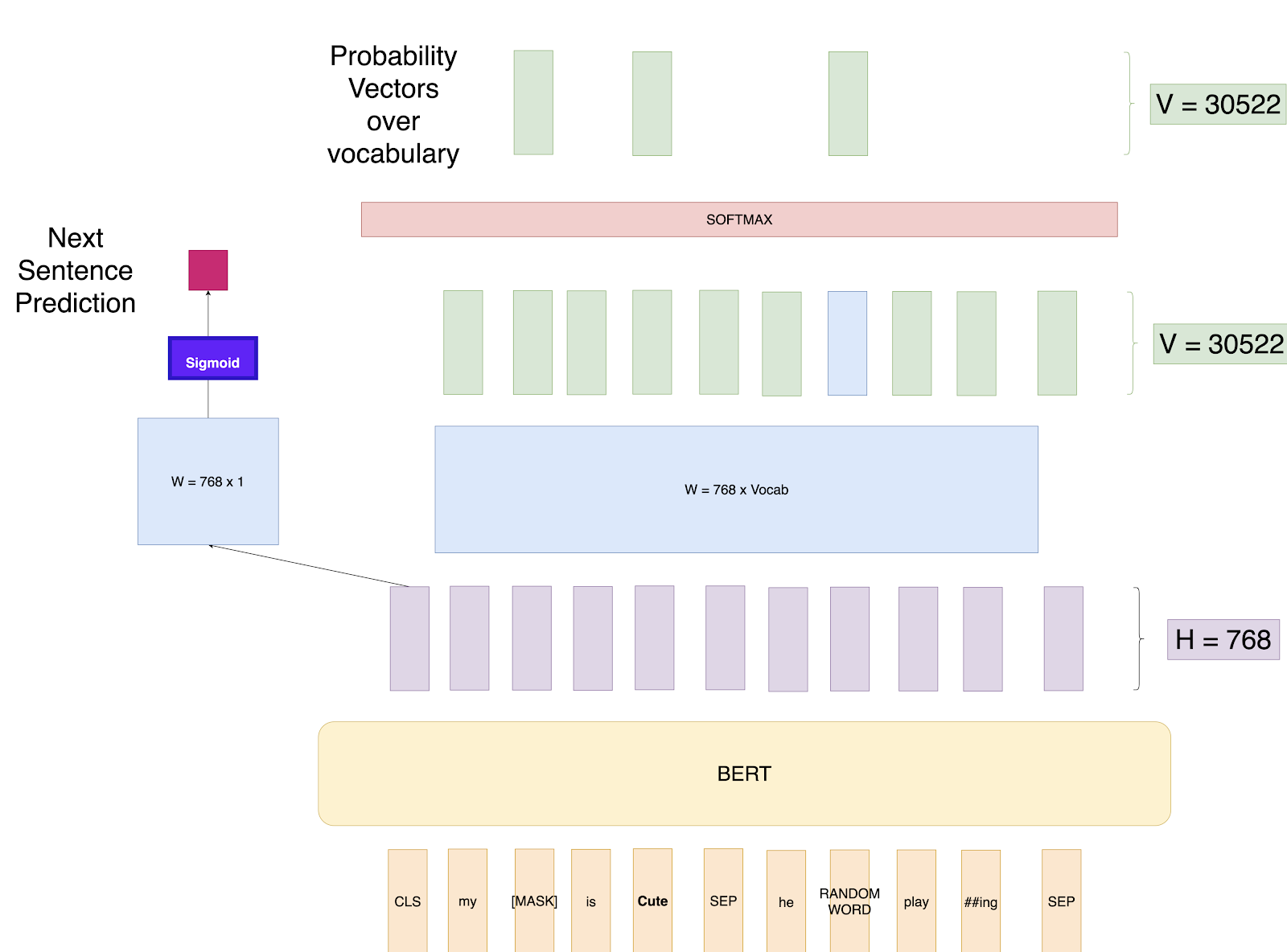So we have got the BERT model now that can provide us with contextual embeddings. How can we use it for various tasks?

### Fine Tuning for Relevant Task

We already have seen how we can use BERT for the classification task by adding a few layers on top of the [CLS] output and fine tuning the weights.BERT Finetuning for Classification

Here is how we can use BERT for other tasks, from the paper: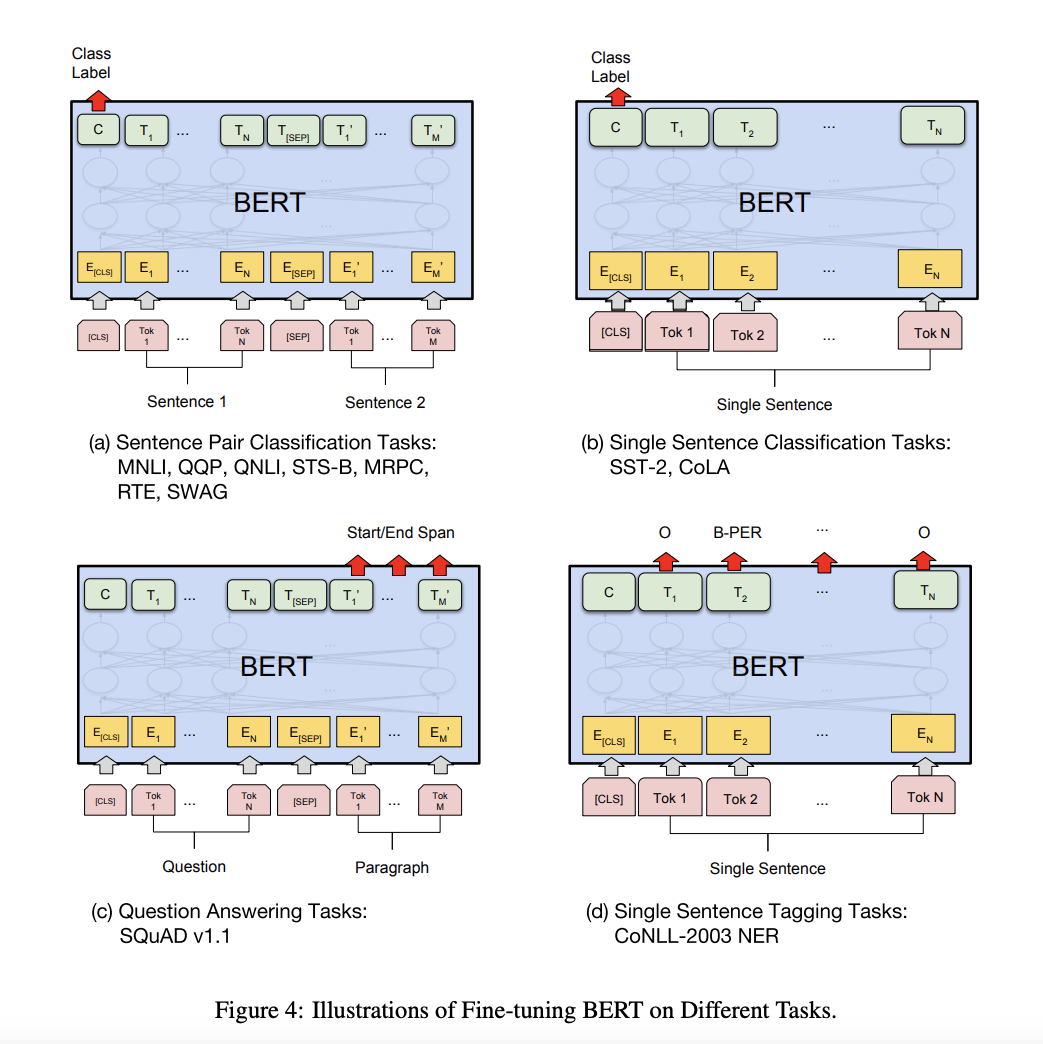Source: BERT Paper

Let’s go through each of them one by one.

1. Sentence Pair Classification tasks — This is pretty similar to the classification task. That is add a Linear + Softmax layer on top of the 768 sized CLS output.
2. Single Sentence Classification Task — Same as above
3. Single Sentence Tagging Task —This is pretty similar to the setup we use while training BERT, just that we need to predict some tags for each token rather than the word itself. For example, for a POS Tagging task like predicting Noun, Verb, or Adjective, we will just add a Linear layer of size (768 x n_outputs) and add a softmax layer on top to predict.
4. Question Answering Tasks — This is the most interesting task and would need some more context to understand how BERT is used to solve it. In this task, we are given a question and a paragraph in which the answer lies. The objective is to determine the start and end span for the answer in the paragraph.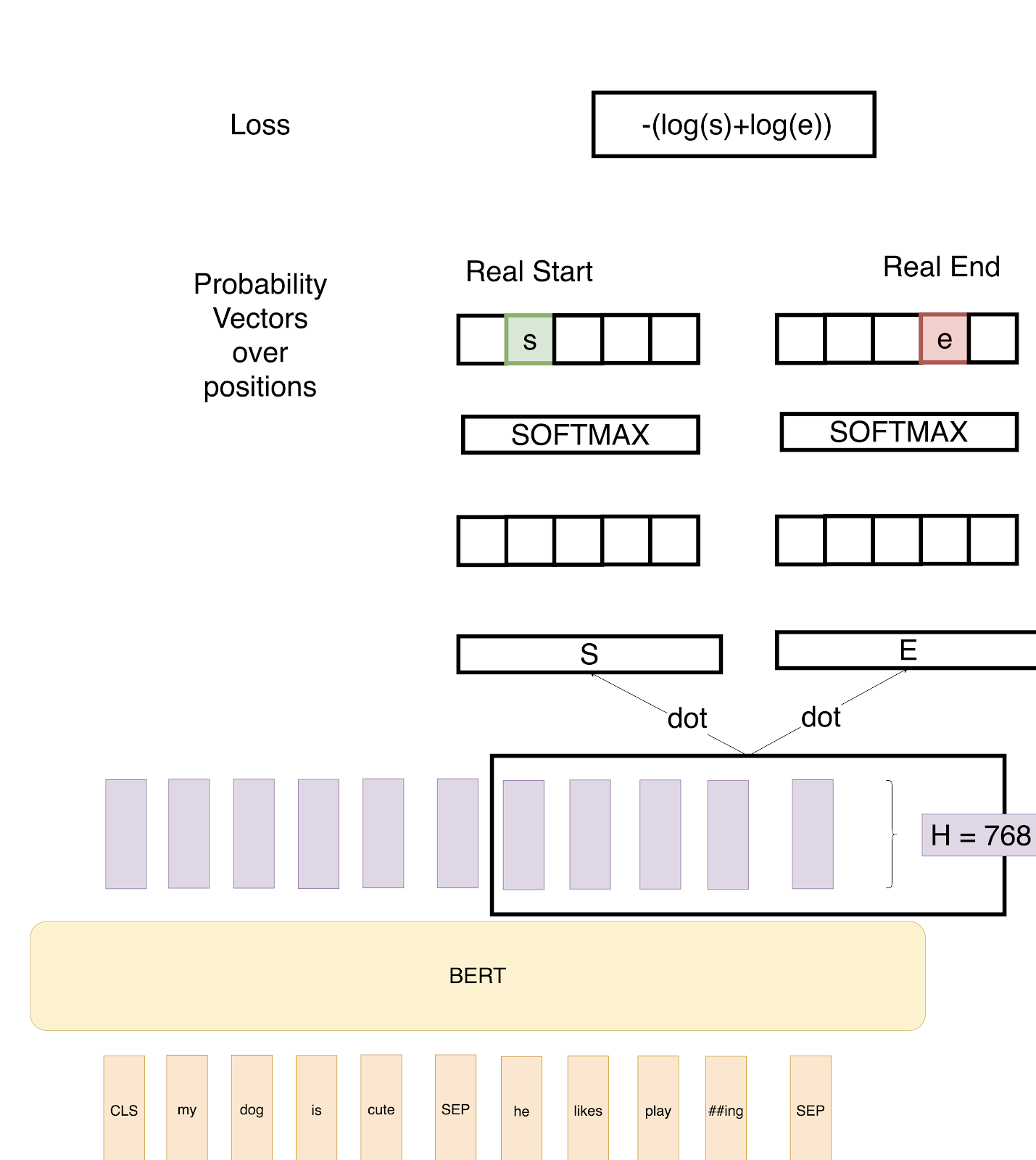So in the above example, we define two vectors S and E (which will be learned during fine-tuning) both having shapes(1x768). We then take a dot product of these vectors with the second sentence’s output vectors from BERT, giving us some scores. We then apply Softmax over these scores to get probabilities. The training objective is the sum of the log-likelihoods of the correct start and end positions. Mathematically, for the Probability vector for Start positions: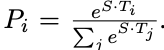where T_weis the word we are focusing on. An analogous formula is for End positions.

To predict a span, we get all the scores — S.T and E.T and get the best span as the span having the maximum Score, that is max(S.T_we+ E.T_j) among all j≥i.

Have any questions about BERT or other AI research involving Transformers?
Contact Exxact Today

### References MentionedDeep Learning

## BERT Transformers – How Do They Work?

### BERT Transformers Are Revolutionary But How Do They Work?

BERT, introduced by Google in 2018, was one of the most influential papers for NLP. But it is still hard to understand. BERT stands for Bidirectional Encoder Representations from Transformers.

In this article, we will go a step further and try to explain BERT Transformers. BERT is one of the most popular NLP models that utilizes a Transformer at its core and which achieved State of the Art performance on many NLP tasks including Classification, Question Answering, and NER Tagging when it was first introduced.

Specifically, we will try to go through the highly influential BERT paperPre-training of Deep Bidirectional Transformers for Language Understanding while keeping the jargon to a minimum.

### What is BERT?

In simple words, BERT is an architecture that can be used for a lot of downstream tasks such as question answering, Classification, NER etc. One can assume a pre-trained BERT as a black box that provides us with H = 768 shaped vectors for each input token(word) in a sequence. Here, the sequence can be a single sentence or a pair of sentences separated by the separator [SEP] and starting with a token [CLS]. We will get into explaining these tokens in more detail in later stages.A very High view of BERT — We get a 768 sized vector for all words in our input sentence.

### What is the Use of a BERT Blackbox?

A BERT model works like how most Deep Learning models for ImageNet work

First, we train the BERT model on a large corpus (Masked LM Task), and then we finetune the model for our own task which could be classification, Question Answering or NER, etc. by adding a few extra layers at the end.

For example, we would train BERT first on a corpus like Wikipedia (Masked LM Task) and then Fine Tune the model on our own data to do a classification task like classifying reviews as negative or positive or neutral by adding a few extra layers. In practice, we just use the output from the [CLS] token for the classification task. So, the whole architecture for fine-tuning looks like this:We just use the [CLS] Token output for classification along with some added Linear and Softmax Layers.

In other similarly published articles on transformers, all Deep Learning is just Matrix Multiplication, where we just introduce a new W layer having a shape of (H x num_classes = 768 x 3) and train the whole architecture using our training data and Cross-Entropy loss on the classification.

We could have also acquired the sentence features through the last layer and then just run a Logistic regression classifier on top or take an average of all the outputs and then run a logistic regression on top. There are many possibilities, and what works best will depend on the data for the task.

In the above example, we explained how you could do Classification using BERT. In pretty much similar ways, one can also use BERT for Question Answering and NER based Tasks. We will get to the architectures used for various tasks in a few sections below.

Interested in an AI workstation that can handle natural language processing?
View deep learning workstations starting at \$3,700

### How is this Different Than Embedding?

This is a great question. Essentially, BERT just provides us with contextual-bidirectional embeddings.

Let’s unpack what that means:

• Contextual: The embeddings of a word are not static. That is, they depend on the context of words around it. So in a sentence like "one bird was flying below another bird", the two embeddings of the word "bird" will be different.
• Bi-Directional: While directional models in the past like LSTM’s read the text input sequentially (left-to-right or right-to-left), the Transformer actually reads the entire sequence of words at once and thus is considered bidirectional.

So, for a sentence like "BERT model is awesome." the embeddings for the word "model" will have context from all the words "BERT", "Awesome", and "is".

### How Does It Work?

Now that we understand the basics, we will divide this section into three major parts: Architecture, Inputs, and Training.

#### 1. Architecture

This is the most simple part to understand if you’re familiar with this post on Transformers. BERT is essentially just made up of stacked up encoder layers.In the paper, the authors have experimented with two models:

• BERT Base: Number of Layers L=12, Size of the hidden layer, H=768, and Self-attention heads, A=12 with Total Parameters=110M
• BERT Large: Number of Layers L=24, Size of the hidden layer, H=1024, and Self-attention heads, A=16 with Total Parameters=340M

#### 2. Training InputsInputs to BERT. Source

We give inputs to BERT using the above structure. The input consists of a pair of sentences, called sequences, and two special tokens: [CLS] and [SEP].

So, in this example, for two sentences “my dog is cute” and “he likes playing”, BERT first uses wordpiece tokenization to convert the sequence into tokens and adds the [CLS] token in the start and the [SEP] token in the beginning and end of the second sentence, so the input is:The wordpiece tokenization used in BERT necessarily breaks words like playing into “play” and “##ing”. This helps in two ways:

• It helps limit the size of Vocabulary as we don’t have to keep the various forms of words like playing, plays, player etc. in our vocabulary.
• It helps us with out-of-vocab words. For example, if plays don’t occur in the vocabulary, we might still have embeddings for play and ##s

Token Embeddings: We then get the Token embeddings by indexing a Matrix of size 30000x768(H). Here, 30000 is the Vocab length after wordpiece tokenization. The weights of this matrix would be learned while training.Token Embeddings come by indexing a matrix of size VocabxH.

Segment Embeddings: For tasks such as question answering, we should specify which segment this sentence is from. These are either all 0 vectors of H length if the embedding is from sentence 1, or a vector of 1’s if the embedding is from sentence 2.Segment embedding are all 0's or all 1’s vector specifying 1st Sentence or 2nd Sentence.

Position Embeddings: These are the embeddings used to specify the position of words in the sequence, the same as we did in the transformer architecture. So we essentially have a constant matrix with some preset pattern. This matrix has the number of columns as 768. The first row of this matrix is the embedding for token [CLS], the second row is embedding for the word “my”, the third row is embedding for the word “dog” and so on.Patterns are used to specify word position.

So the Final Input given to BERT is Token Embeddings + Segment Embeddings + Position Embeddings.

We finally reach the most interesting part of BERT here, as this is where most of the novel concepts are introduced. We will try to explain these concepts by going through various architecture attempts and finding faults with each of them to arrive at the final BERT architecture.

Attempt 1: So, for example, if we set up BERT training as below:Attempt 1: Predict all words.

We try to predict each word of the input sequence using our training data with Cross-Entropy loss.

Can you guess a potential problem with this approach?

The problem is that the learning task is trivial.

The network knows beforehand what it has to predict, and it can thus easily learn weights to reach a 100% classification accuracy.

Attempt 2: Masked LM: This starts the beginning of the paper's approach to overcoming the previous approach's problem. We mask 15% random words in each training input sequence and just predict output for those words. In Pictorial terms:Attempt 2: Predict only masked words.

Thus the loss gets calculated only over masked words. So now the model learns to predict words it hasn’t seen while seeing all the context around those words.

Please note, we have masked 3 words here even when we should mask just 1 word as 15% of 8 is 1 to explain in this toy example.

Can you find the problem with this approach?

This model has essentially learned that it should predict good probabilities for only the [MASK] token. That is at the prediction time or at the fine-tuning time when this model will not get [MASK] as input; the model won’t predict good contextual embeddings.

Attempt 3: Masked LM with random Words:

In this attempt, we will still mask 15% of the positions. But we will replace any word in 20% of those masked tokens by some random word. We do this because we want to let the model know that we still want some output when the word is not a [MASK] token. So if we have a sequence of length 500, we will mask 75 tokens(15% of 500), and in those 75 tokens, 15 tokens(20 % of 75) would be replaced by random words. Pictorially, here we replace some of the masks by random words.Attempt 3: Predict masked words and Random Words.

Advantage: The network will still work with any word now.

Problem: The network has learned that Input Word is never equal to the Output word. That is the output vector at the position of “random word” would never be “random word.”

To solve this problem, the authors suggest the below training setup.

The training data generator chooses 15% of the token positions at random for prediction. If the i-th token is chosen, we replace the i-th token with (1) the [MASK] token 80% of the time (2) a random token 10% of the time (3) the unchanged i-th token 10% of the time

So if we have a sequence of length 500, we will mask 75 tokens(15% of 500), and in those 75 tokens, 7 tokens(10 % of 75) would be replaced by random words, and 7 tokens (10% of 75) will be used as it is. Pictorially, we replace some of the masks with random words and replace some of the masks by actual words.So, now we have the best setup where our model doesn’t learn any unsavory patterns.

But what if we keep only Mask + Unmask Setup? The model will learn that whenever the word is present, just predict that word.

From the BERT paper:

Many important downstream tasks such as Question Answering (QA) and Natural Language Inference (NLI) are based on understanding the relationship between two sentences, which is not directly captured by language modeling. In order to train a model that understands sentence relationships, we pre-train for a binarized next sentence prediction task that can be trivially generated from any monolingual corpus.

So, now we understand the Masked LM task, BERT Model also has one more training task which goes in parallel while Training Masked LM task. This task is called Next Sentence Prediction (NSP). So while creating the training data, we choose the sentences A and B for each training example such that 50% of the time B is the actual next sentence that follows A (labelled as IsNext), and 50% of the time it is a random sentence from the corpus (labelled as NotNext). We then use the CLS token output to get the binary loss which is also back propagated through the network to learn the weights.So we have got the BERT model now that can provide us with contextual embeddings. How can we use it for various tasks?

### Fine Tuning for Relevant Task

We already have seen how we can use BERT for the classification task by adding a few layers on top of the [CLS] output and fine tuning the weights.BERT Finetuning for Classification

Here is how we can use BERT for other tasks, from the paper:Source: BERT Paper

Let’s go through each of them one by one.

1. Sentence Pair Classification tasks — This is pretty similar to the classification task. That is add a Linear + Softmax layer on top of the 768 sized CLS output.
2. Single Sentence Classification Task — Same as above
3. Single Sentence Tagging Task —This is pretty similar to the setup we use while training BERT, just that we need to predict some tags for each token rather than the word itself. For example, for a POS Tagging task like predicting Noun, Verb, or Adjective, we will just add a Linear layer of size (768 x n_outputs) and add a softmax layer on top to predict.
4. Question Answering Tasks — This is the most interesting task and would need some more context to understand how BERT is used to solve it. In this task, we are given a question and a paragraph in which the answer lies. The objective is to determine the start and end span for the answer in the paragraph.So in the above example, we define two vectors S and E (which will be learned during fine-tuning) both having shapes(1x768). We then take a dot product of these vectors with the second sentence’s output vectors from BERT, giving us some scores. We then apply Softmax over these scores to get probabilities. The training objective is the sum of the log-likelihoods of the correct start and end positions. Mathematically, for the Probability vector for Start positions:where T_weis the word we are focusing on. An analogous formula is for End positions.

To predict a span, we get all the scores — S.T and E.T and get the best span as the span having the maximum Score, that is max(S.T_we+ E.T_j) among all j≥i.

Have any questions about BERT or other AI research involving Transformers?
Contact Exxact Today